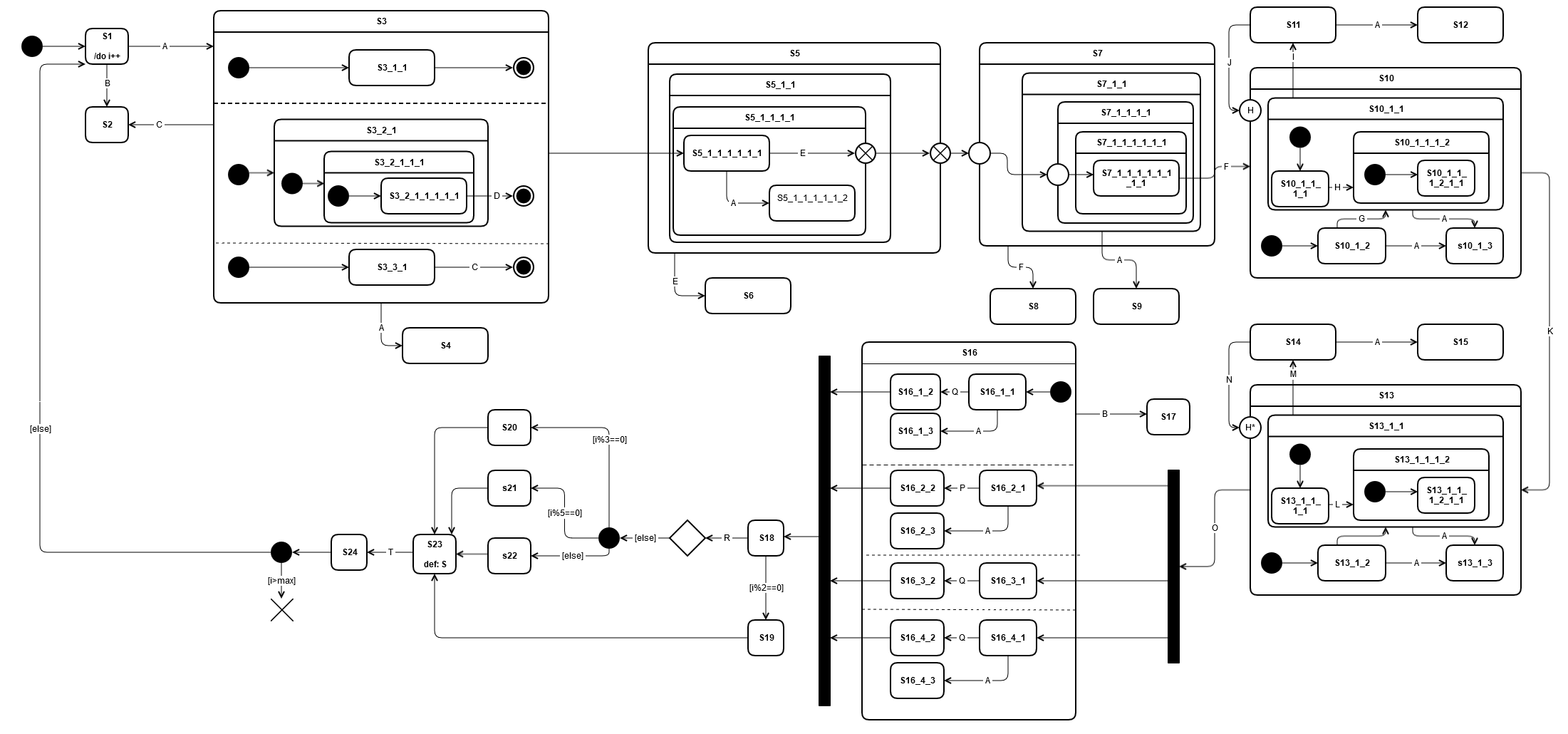# Forty two (42)

'Forty two' is the name of a state machine example that contains all the different kind of vertices. We use it for performance and endurance tests.

The UML diagram of the 'forty two' state machine:This state machine is encapsulated inside a class named 'forty_two'. The following paragraphs describe the construction of the class that owns and operates the state machine.

# Constructor of the 'forty_two'

`forty_two::forty_two( ``const`` sxy::uint32_t _max_iterations )`
` : state_machine_( build_state_machine() ),`
` iterations_(0),`
` max_iterations_( _max_iterations )`
`{`
` SX_ASSERT( check_state_machine(), ``"State machine has defects!"`` );`
` run();`
`}`

In the initialization list, we create the state machine and move it in the class member 'state_machine_'. Also, the iterations counter will be set to zero and the maximum iteration will be set to value given by the user.

One iteration means one run through the whole state machine form State 1 to State 1.

# run()

In the run method, a set of events from 'A' to 'T' will be fired, one after another. The sequence of the events will be processed and after 'T' is fired, if the maximum iteration number is not reached, the state machine will process another set of events from 'A' to 'T'.

If the macro 'SX_NO_LOGGING' is defined, then there will be no logging. In case the logger is enabled and the 'Y_PROFILER' macro is defined, then the number of processed events will be logged as well.

`bool`` starting_success = state_machine_->run();`
`SX_ASSERT( starting_success, ``"State machine was not started!"`` );`
`for``( uint32_t iteration = 0; iteration < max_iterations_; ++iteration )`
`{ `
` state_machine_->fire_event( event_A::create() );`
` state_machine_->fire_event( event_B::create() );`
` state_machine_->fire_event( event_C::create() );`
` state_machine_->fire_event( event_D::create() );`
` state_machine_->fire_event( event_E::create() );`
` state_machine_->fire_event( event_F::create() );`
` state_machine_->fire_event( event_G::create() );`
` state_machine_->fire_event( event_H::create() );`
` state_machine_->fire_event( event_I::create() );`
` state_machine_->fire_event( event_J::create() );`
` state_machine_->fire_event( event_K::create() );`
` state_machine_->fire_event( event_L::create() );`
` state_machine_->fire_event( event_M::create() );`
` state_machine_->fire_event( event_N::create() );`
` state_machine_->fire_event( event_O::create() );`
` state_machine_->fire_event( event_P::create() );`
` state_machine_->fire_event( event_Q::create() );`
` state_machine_->fire_event( event_R::create() );`
` state_machine_->fire_event( event_S::create() );`
` state_machine_->fire_event( event_T::create() );`
`}`
`state_machine_->stop();`

# Building the 'forty two' state machine

In the 'build_state_machine' method the state machine will be created and all elements will be stuck together. The transitions and behaviors will also be created in here.

There are two kinds of pseudostates: ones that belong to a region and ones that belong to a composite state. The pseudostates that belong to regions are initial pseudostates, terminate pseudostates, choices, junctions, forks and joins. Entry and exit points, shallow and deep histories belong to a composite state.

A region class has methods for adding all associated pseudostates and states. Here are some code examples from the 42 example:

`initial_pseudostate& i1 = main_region.add_initial_pseudostate( ``"initial pseudostate 1"`` );`
`terminate_pseudostate& terminate_pseudostate_1 = main_region.add_terminate_pseudostate( ``"terminate pseudostate 1"`` );`
`choice& choice1 = main_region.add_choice( ``"choice 1"`` );`
`junction& junction1 = main_region.add_junction( ``"junction 1"`` );`
`join& join1 = main_region.add_join( ``"join 1"`` );`
`fork& fork1 = main_region.add_fork( ``"fork 1"`` );`

`simple_state& s2 = main_region.add_simple_state( ``"s2"`` );`
`composite_state& s3 = main_region.add_composite_state( ``"s3"`` );`
`final_state& final2 = r3_2.add_final_state( ``"final state 2"`` );`

A composite state has methods for adding entry and exit points as well as shallow and deep histories. The following lines are showing how to add them:

`entry_point& entry1 = s7_1_1_1_1.add_entry_point( ``"entry1"`` );`
`exit_point& exit1 = s5_1_1_1_1.add_exit_point( ``"exit1"`` );`
`shallow_history& shallow_history1 = s10.add_shallow_history( ``"shallow history 1"`` );`
`deep_history& deep_history1 = s13.add_shallow_history( ``"deep history 1"`` );`

It is possible to add a free function or a class method as a transition's guard. The easiest way to do this is by using the predefined macros that are described on the assembly page. In the forty two example, Y_GUARD_METHOD2 is used:

`l_state_machine->add_transition( sxy::Y_COMPLETION_EVENT_ID, choice1, s19,`
` Y_GUARD_METHOD2( ``this``, &forty_two::check_iterations_divided_by_2 ) );`

The methods used for the guards have to return a boolean value. This value indicates if the guard lets the event pass or not.

The method used by the guard above looks like this:

`bool`` forty_two::check_iterations_divided_by_2() ``const`
`{`
` ``return`` ( iterations_ % 2 == 0 );`
`}`

Adding a behavior is also as simple as a call of a predefined macro. Again the predefined macros are described on the assembly page. In the forty two example just one state has a method as a behavior.

Here is the piece of code that does this utilizing the macro Y_BEHAVIOR_METHOD2:

`sxy::simple_state& s1 = main_region.add_simple_state( ``"s1"``, `
` Y_BEHAVIOR_METHOD2( ``this``, &forty_two::increment_iterations ) );`

The methods for behaviors can return a value of any type as it is ignored by the state machine.

The method used for the behavior above looks like:

`void`` forty_two::increment_iterations()`
`{`
` ++iterations_;`
` SX_LOG( hermes::log_level::LL_DEBUG, ``"iterator incremented to %"``, iterations_ );`
`}`

The source code can be found on GitHub.